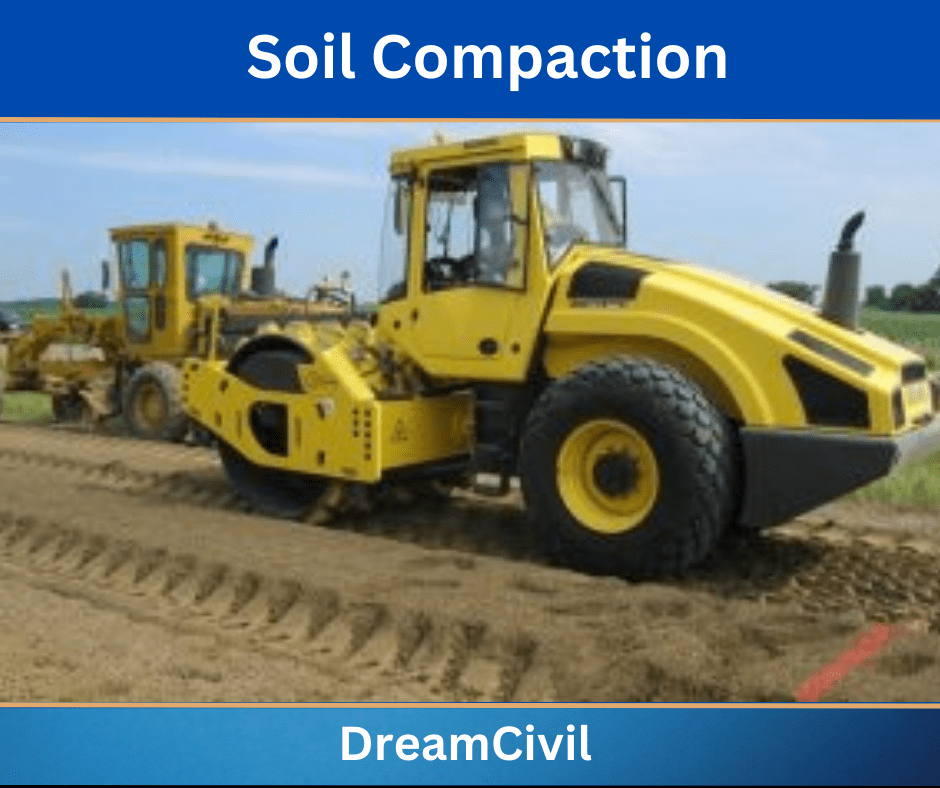Tuesday, October 3, 2023

# Soil Compaction Numerical | Soil Mechanics Numericals

Before solving the soil compaction numerical, we need to know the formula and use of it.

### 1. Formula

 Dry unit weight (γd) = Gγw/ 1+e

where,

e = Void ratio

 Volume of borrow pit soil required = (γw)embankment x V1/ (γd)borrow pit

Where,

V1 = Volume of finished embankement

 Degree of Saturation (Sr)= (w x G)/e

Where,

G = Specific Gravity

 Number of truck trips = V2/ Per truck loads

Where,

V2= Volume of borrow pit soil required.

 Water Content = Mwet – Mdry / Mdry

where,

Mdry = Mass of dry soil.

Mwet = Mass of wet soil.

 In-situ dry density of soil(ρdry) = ρt/ 1+w

Where,

ρt = In-situ bulk density of soil.

w= water content### 2. Soil Compaction Numerical

1. An embankment for a highway 30 m wide and 1.5 m compacted thickness is to be constructed from sandy soil trucked from a borrow pit. The water content of the sandy soil in the borrow pit is 15% and its void ratio is 0.69. The specification requires the embankment compacted to a dry unit weight of 18 kN/m3. determine for 1 km length of embankments that following,

i) The dry unit weight of sandy soil from the borrow pit required to construct the embankment.

ii) The number of 10 m3 truckloads of sandy soil required for the construction.

iii) The degree of saturation of the sandy soil in situ.

Solution:

Assuming that the specific gravity of soil (G) = 2.70

i) The borrow pit’s dry unit weight, γd = G γw/ (1+e)

= 2.7 x 9.81/ (1+0.69)

= 15.67 kN/m3

The volume of the finished embankment (V1)= 30 m x 1.5 m x (1 km long)

= 45 x 103 m3

Volume of borrow pit soil required = (γw)embankment x V1/ (γd)borrow pit

= 18 x 45 x103 / 15.67

= 51691.13 m3

Number of truck trips = V2 / Per truck loads

= 51691.13/10

iii) Degree of Saturation (Sr) = (w x G) / e

Sr = (0.15 x 2.70) / 0.69

Sr = 0.59 = 59 %

 Read Also: Quantity of Water Numerical

2. The maximum dry unit weight of a compacted soil mass is found to be 18 kN/m3 with optimum water content being 15%. Find the values of porosity and degree of saturation of this compacted soil. Also, find the value of the maximum dry unit weight on the zero air void line at that optimum water content. Take the specific gravity of soil solid as 2.70.

Solution:

Given that;

Maximum dry unit weight (γd)max = 18 kN/m3

Optimum water content (wopt) = 15% = 0.15

Specific gravity of soil solid (G)= 2.70

Now,

γd = Gγw/(1+ wG/Sr)

18 = 2.70 x 9.81 / (1+ 0.15 x 2.70 / Sr)

Sr = 0.15 x 2.70 /0.4715

Sr = 85.90%

Again, we have

Sr x e = wG

or, e = 0.15 x 2.70/ 0.859 = 0.471

and, n= e/ (1+e)

n = 0.471 / (1+0.471)

At zero air void line; we have,

γd = Gγw/ (1+wG)

= 2.70 x 9.81/ 1+(0.15 x 2.70)

= 18.85 kN/m3

 Read Also: Hardness and Alkalinity Numerical

3. In the construction of a road, the compaction specification required was 95% off proctor maximum dry density at field moisture content within 2% of the optimum moisture content. The maximum dry density and optimum moisture content obtained in the laboratory from the standard proctor test were 1.95 Mg/m3 and 13.5% respectively. A site engineer conducted a sand cone test in two locations and obtained the following results.

 Location no. Mass of soil removed (gm) Mass of sand used (gm) Wet Dry 1 43.86 38.46 39.51 2 37.38 32.21 32.39

The density of sand used was 1.86 Mg/m3. Check whether the specification was satisfied or not.

Solution:

Maximum dry unit weight (ρd)max = 1.95 Mg/m3 = 1.95 gm/cm3

Optimum water content (wopt) = 13.5%

Density of sand (ρsand) = 1.86 Mg/m3 = 1.86 gm/cm3

Calculation results of sand cone test

(i) For location 1

Bulk density of sand = 1.86 Mg/m3

Mass of sand used= 39.51

The volume of sand required to fill up the pit is given by;

Volume of pit(V) = M/ρ

= 39.51/1.86

= 21.24 cm3

But, the mass of soil excavated from the pit= 43.86 gm

In-situ bulk density of soil (ρt) = 43.86/21.24

= 2.06 gm/cm3

The water content of soil (w) = (Mwet – Mdry) / Mdry

= (43.86-38.46) / 38.46

= 0.1404

= 14.04%

Now, In-situ dry density of soil (ρdry) = ρt / (1+w)

= 2.06/(1+ 0.1404)

= 1.81 gm/cm3

ii) For location 2:

The volume of sand required to fill the pit (V) = M/ρ

= 32.39/1.86

= 17.41 cm3

The in-situ density of soil (ρt) = 37.38/17.41

= 2.15gm/cm3

Water content (w)= (37.38-32.21)/32.21

= 0.1605

= 16.05%

Now,

In-situ dry density of soil (ρdry) = ρt / (1+w)

= 2.15/(1+ 0.1605)

= 1.85 gm/cm3

Thus, actual field results are the average of values obtained from locations 1 and 2;

Dry density (ρdry) = (1.81+1.85) / 2

Water content (w) = (0.1404+0.1605) / 2

= 0.1504

=15.04%

Compare specification with sand cone test

Minimum dry unit required= 0.95 x 1.95 = 1.8525 gm/cm3

Field water content = (13.5 +2) /100

= 0.27

Thus, the field water content should be within 13.5±0.27.

The sand cone test gives a dry density of 1.83 gm/cm3 and water content of 15.04%.

After compacting these data, it is clear that the specification was not satisfied.

### 3. References

1. Content Filter & Authenticity Checking Team, Dream Civil International

(Our team checks every content & detail to maintain quality. )He is a founder and lead author of Dream Civil International and his civil engineering research articles has been taken as source by world's top news and educational sites like USA Today, Time, The richest, Wikipedia, etc.
Latest Articles

Related Articles